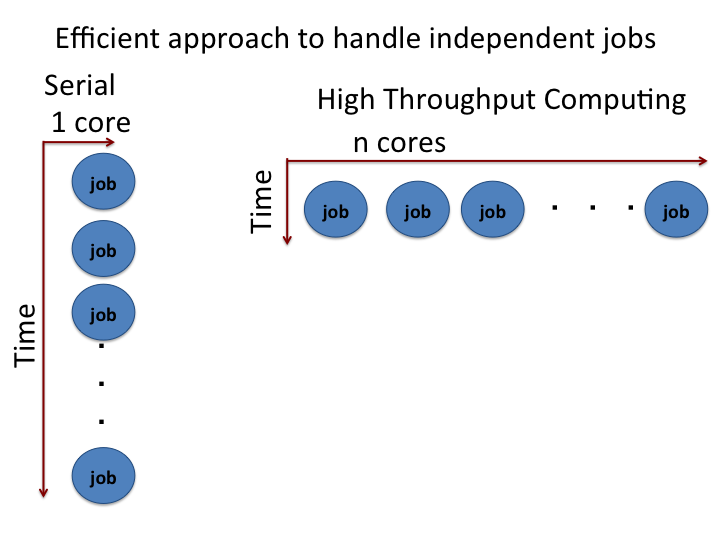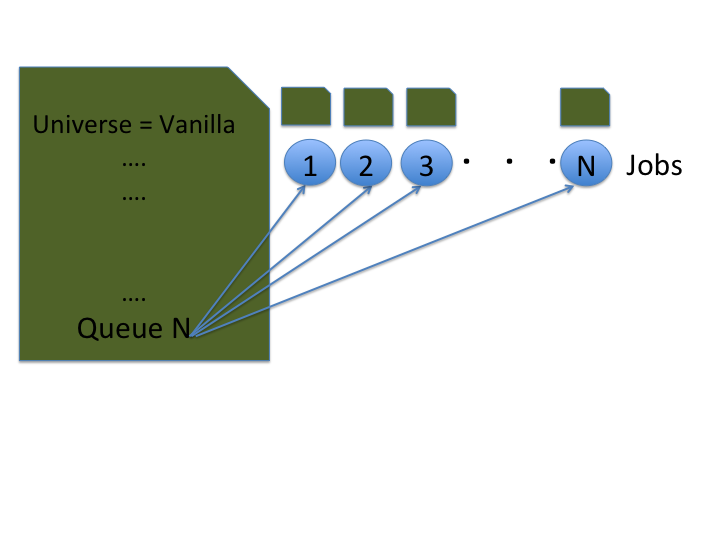## Overview

Many large scale computations require the ability to process multiple jobs concurrently. Consider the extensive sampling done for a multi-dimensional Monte Carlo integration, parameter sweep for a given model or molecular dynamics simulation with several initial conditions. These calculations require submitting many jobs. About a million CPU hours per day are available to OSG users on an opportunistic basis. Learning how to scale up and control large numbers of jobs is essential to realize the full potential of distributed high throughput computing on the OSG.The HTCondor's `queue` command can run multiple jobs from a single job description file. In this tutorial, we will see how to scale up the calculations for a simple python example using the HTCondor’s queue command.

Once we understand the basic HTCondor script to run a single job, it is easy to scale up.

Obtain the example files via the `tutorial` command,

``````\$ tutorial ScalingUp-Python
\$ cd tutorial-ScalingUp-Python
``````

Inside the `tutorial-ScalingUp-python` directory, all the required files are available. This includes the sample python program, job description file and executable files.

## Python script and the optimization function

Let us take a look at our objective function that we are trying to optimize.

``````    f = (1 - x)**2 + (y - x**2)**2
``````

This a two dimensional Rosenbrock function. Clearly, the minimum is located at (1,1). The Rosenbrock function is one of the test functions used to test the robustness of an optimization method.Here, we are going to use the brute force optimization approach to evaluate the two dimensional Rosenbrock function on grids of points. The boundary values for the grid points are randomly assigned inside the python script. However, these default values may be replaced by user supplied values.

To run the calculations with the random boundary values, the script is executed without any argument:

``````module load py-scipy/1.1.0-py3.7
python rosen_brock_brute_opt.py
``````

To run the calculations with the user supplied values, the script is executed with input arguments:

``````python rosen_brock_brute_opt.py x_low x_high y_low y_high
``````

where x_low and x_high are low and high values along x direction, and y_low and y_high are the low and high values along the y direction.

For example, the boundary of x direction is (-3, 3) and the boundary of y direction is (-2, 3).

``````python rosen_brock_brute_opt.py  -3 3 -2 2
``````

sets the boundary of x direction to (-3, 3) and the boundary of y direction to (-2, 3).

The directory `Example1` runs the python script with the default random values. The directories `Example2`, and `Example3` deal with supplying the boundary values as input arguments.

## Execution Script

Let us take a look at the execution script, `scalingup-python-wrapper.sh`

``````#!/bin/bash

module load py-scipy/1.1.0-py3.7

python3 ./rosen_brock_brute_opt.py  \$1 \$2 \$3 \$4
``````

The wrapper loads the the relevant module and then executes the python script `rosen_brock_brute_opt.py`. The python script takes four argument but they are optional. If we don't supply these optional arguments, the values are internally assigned.

## Submitting Jobs ConcurrentlyNow let us take a look at job description file.

``````cd Example1
cat ScalingUp-PythonCals.submit
``````

If we want to submit several jobs, we need to track log, out and error files for each job. An easy way to do this is to add the `\$(Cluster)` and `\$(Process)` variables to the file names. You can see this below in the names given to the standard output, standard error and HTCondor log files:

``````executable = ../scalingup-python-wrapper.sh

transfer_input_files = ../rosen_brock_brute_opt.py

log = Log/job.\$(Cluster).\$(Process).log
output = Log/job.\$(Cluster).\$(Process).out
error = Log/job.\$(Cluster).\$(Process).err

requirements = OSGVO_OS_STRING =?= "RHEL 7" && HAS_MODULES =?= True
request_cpus = 1
request_memory = 1 GB
request_disk = 1 GB

queue 10
``````

Note the `queue 10`. This tells Condor to queue 10 copies of this job as one cluster.

Let us submit the above job

``````\$ condor_submit ScalingUp-PythonCals.submit
Submitting job(s)..........
10 job(s) submitted to cluster 329837.
``````

Apply your `condor_q` knowledge to see this job progress. After all jobs finished, execute the `post_script.sh` script to sort the results.

``````./post_script.sh
``````

Note that all ten jobs will have run with random arguments because we did not supply any from the submit file. What if we wanted to supply those arguments so that we could reproduce this analysis if needed? The next example shows how to do this.

## Providing Different Inputs to Jobs

In the previous example, we did not pass any argument to the program and the program generated random boundary conditions. If we have some guess about what could be a better boundary condition, it is a good idea to supply the boundary condition as arguments.

It is possible to use a single file to supply multiple arguments. We can take the job description file from the previous example, and modify it to include arguments. The modified job description file is available in the `Example2` directory. Take a look at the job description file `ScalingUp-PythonCals.submit`.

``````\$ cd ../Example2
\$ cat ScalingUp-PythonCals.submit

executable = ../scalingup-python-wrapper.sh
arguments = \$(x_low) \$(x_high) \$(y_low) \$(y_high)

transfer_input_files = ../rosen_brock_brute_opt.py

log = Log/job.\$(Cluster).\$(Process).log
output = Log/job.\$(Cluster).\$(Process).out
error = Log/job.\$(Cluster).\$(Process).err

requirements = OSGVO_OS_STRING =?= "RHEL 7" && HAS_MODULES =?= True
request_cpus = 1
request_memory = 1 GB
request_disk = 1 GB

queue x_low x_high y_low y_high from job_values.txt
``````

A major part of the job description file looks same as the previous example. The main difference is that the addition of `arguments` keyword, which looks like this:

``````arguments = \$(x_low) \$(x_high) \$(y_low) \$(y_high)
``````

The given arguments `\$(x_low)`, `\$(x_high)`, etc. are actually variables that represent the values we want to use. These values are set in the queue command at the end of the file:

``````queue x_low x_high y_low y_high from job_values.txt
``````

Take a look at job_values.txt:

``````\$ cat job_values.txt

-9 9 -9 9
-8 8 -8 8
-7 7 -7 7
-6 6 -6 6
-5 5 -5 5
-4 4 -4 4
-3 3 -3 3
-2 2 -2 2
-1 1 -1 1
``````

The submit file's `queue` statement will read in this file and assign each value in a row to the four variables shown in the `queue` statement. Each row corresponds to the submission of a unique job with those four values.

Let us submit the above job to see this:

``````\$ condor_submit ScalingUp-PythonCals.submit
Submitting job(s)..........
9 job(s) submitted to cluster 329840.
``````

Apply your `condor_q` knowledge to see this job progress. After all jobs finished, execute the `post_script.sh` script to sort the results.

``````./post_process.sh
``````

## Another Example of Different Inputs

In the previous example, we split the input information into four variables that were included in the `arguments` line. However, we could have set the `arguments` line directly, without intermediate values. This is shown in Example 3:

``````\$ cd ../Example3
\$ cat ScalingUp-PythonCals.submit

executable = ../scalingup-python-wrapper.sh

transfer_input_files = ../rosen_brock_brute_opt.py

log = Log/job.\$(Cluster).\$(Process).log
output = Log/job.\$(Cluster).\$(Process).out
error = Log/job.\$(Cluster).\$(Process).err

requirements = OSGVO_OS_STRING =?= "RHEL 7" && HAS_MODULES =?= True
request_cpus = 1
request_memory = 1 GB
request_disk = 1 GB

queue arguments from job_values.txt
``````

Here, `arguments` has disappeared from the top of the file because we've included it in the queue statement at the end. The `job_values.txt` file has the same values as before; in this syntax, HTCondor will submit a job for each row of values and the job's arguments will be those four values.

Let us submit the above job

``````\$ condor_submit ScalingUp-PythonCals.submit
Submitting job(s)..........
9 job(s) submitted to cluster 329839.
``````

Apply your `condor_q` and `connect watch` knowledge to see this job progress. After all jobs finished, execute the `post_script.sh` script to sort the results.

``````./post_process.sh
``````

This page was updated on Mar 18, 2021 at 17:36 from tutorials/tutorial-ScalingUp-Python/README.md.